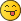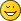# LDraw.org Discussion Forums

Full Version: Help calculating piston rod angle
You're currently viewing a stripped down version of our content. View the full version with proper formatting.
I can't quite figure out how to calculate the angle and vertical distance of a piston rod on a rotated crankshaft, specifically using LDCad's Selection Info tool. Here's a diagram:
[attachment=4054]

So the crankshaft has been rotated -49.5 degrees. The piston rod (A-B) will move up the Y-axis, but I don't know that distance (it's slightly less than the current Y distance between A and C). Also, point B will remain at its current X position but point A will rotate around point B to connect with the axle at point C. The angle of that rotation is the second thing I don't know.

No doubt this has been tackled many times before, but I'd like to know the precise way to figure it out (rather than downloading an already finished model). It's either slightly more difficult, or vastly simpler than I realize.(And I do know about the piston placement script in LDCad, but AFAIK it doesn't recognize these old-style piston parts or brick-built cylinders.)

Again, the two values I need to find are:
• The distance to move the piston rod along the Y-axis, and
• The angle to rotate point A around point B so that it connects with axle C
(2019-09-26, 3:59)N. W. Perry Wrote: [ -> ]I can't quite figure out how to calculate the angle and vertical distance of a piston rod on a rotated crankshaft, specifically using LDCad's Selection Info tool. Here's a diagram:

So the crankshaft has been rotated -49.5 degrees. The piston rod (A-B) will move up the Y-axis, but I don't know that distance (it's slightly less than the current Y distance between A and C). Also, point B will remain at its current X position but point A will rotate around point B to connect with the axle at point C. The angle of that rotation is the second thing I don't know.

No doubt this has been tackled many times before, but I'd like to know the precise way to figure it out (rather than downloading an already finished model). It's either slightly more difficult, or vastly simpler than I realize.(And I do know about the piston placement script in LDCad, but AFAIK it doesn't recognize these old-style piston parts or brick-built cylinders.)

Again, the two values I need to find are:
• The distance to move the piston rod along the Y-axis, and
• The angle to rotate point A around point B so that it connects with axle C

There is a feature in LDCad which may help you do this automatically. See the following image:If you select the three points you are interested in, then right mouse click a part, the "Selection" menu pops up. At the bottom of the menu, there is a menu item "Selection info". Click it and a number of options are shown. For example, to get the angles shown in my diagram, I have "Show Info" set to yes, "Show distance" set to no and "Show angles" set to yes.

Try this and see if you can get the information you are looking for.

Regards,

David
(2019-09-26, 7:34)David Manley Wrote: [ -> ]There is a feature in LDCad which may help you do this automatically. See the following image:

[image snipped]

If you select the three points you are interested in, then right mouse click a part, the "Selection" menu pops up. At the bottom of the menu, there is a menu item "Selection info". Click it and a number of options are shown. For example, to get the angles shown in my diagram, I have "Show Info" set to yes, "Show distance" set to no and "Show angles" set to yes.

Try this and see if you can get the information you are looking for.

Yep, that's the same selection info tool I mentioned. Trouble is, I can't figure out quite how to use its features to get the precise measurements I need, because I don't yet have all three of the points I'm interested in. Looking at my diagram, I can't just take the angle of ABC, because A and B will have moved up the Y axis to a new position, which I don't know. So the angle I need is A'B'C, and first I need to find A' (and by extension, B').

Alternatively, if I knew the angle, I could get the new Y position perfectly easily by using part snapping.
(2019-09-26, 13:20)N. W. Perry Wrote: [ -> ]Yep, that's the same selection info tool I mentioned. Trouble is, I can't figure out quite how to use its features to get the precise measurements I need, because I don't yet have all three of the points I'm interested in. Looking at my diagram, I can't just take the angle of ABC, because A and B will have moved up the Y axis to a new position, which I don't know. So the angle I need is A'B'C, and first I need to find A' (and by extension, B').

Alternatively, if I knew the angle, I could get the new Y position perfectly easily by using part snapping.
Yes, you need to do a bit of trigonometry here...
[attachment=4055]
We want to turn the piston arm so that its tip is at the distance between red pin and initial axis of arm (vertical here). Since I put the gear on the origin, this distance is directly pin X coordinates (13.856), but you can also derive it in the general case from LDCad measurements as sin(16.10°)*49.96. Note that the blue parts are just helpers parts to get the right measurements points.
Once we get this value, we calculate piston arm rotation angle as arcsin(13.856/60)=13.35°. Rotate the arm by this value and use snapping on red pin to correctly position the pistion arm...
(2019-09-26, 14:09)Philippe Hurbain Wrote: [ -> ]Yes, you need to do a bit of trigonometry here...
Philo is right, you can't use the tool directly because you would need to know the final coordinates for a,b and c to get to an angle you don't need anymore in that situation.

I would solve it like so (almost identical to Philo's solution but without the use of the tool):

-take the x distance between a and c -> lx
-take the piston length (b-a=60) -> lp
-cos(cc)=lx/lp
-snap the piston using a to c
-rotate the piston over c using angle a 90-cc angle
-snap the piston head to b
(2019-09-26, 14:09)Philippe Hurbain Wrote: [ -> ]Yes, you need to do a bit of trigonometry here...

We want to turn the piston arm so that its tip is at the distance between red pin and initial axis of arm (vertical here). Since I put the gear on the origin, this distance is directly pin X coordinates (13.856), but you can also derive it in the general case from LDCad measurements as sin(16.10°)*49.96. Note that the blue parts are just helpers parts to get the right measurements points.
Once we get this value, we calculate piston arm rotation angle as arcsin(13.856/60)=13.35°. Rotate the arm by this value and use snapping on red pin to correctly position the pistion arm...

Ah, yes, that's what I was afraid of: the 't' word would come into play!OK, so, since I have to do this again a few times, let me try putting this into a sequence that makes sense to me:
• We need to know the "x" distance from A to C, which forms an opposite triangle leg to angle B.
• We also know the hypotenuse of that triangle (BC), and we know angle B, so we can use sine to solve for "x" because sine is the ratio of opposite (x) to hypotenuse (BC); thus:
• sin(B) = x / (BC) and sin(B)*(BC) = x
• Once the piston rod is rotated, it will become the hypotenuse (B'C) of a new triangle, with a known length of 60.
• x is still the opposite leg to a new angle B', which we need to find. Since we want an angle instead of a length, we use the inverse of sine, or arcsin; thus:
• arcsin( x / B'C ) = B' or arcsin( x / 60 ) = B'
• Rotate the piston rod around point B by angle B', then use part snapping to move the rotated point A onto point C (and consequently, point B onto point B').
To get angle B' using Selection Info, type the following into a calculator:
(angle B) [sin] * (distance B-C) / 60 = [sin-1] =

How'd I do?
(2019-09-27, 5:44)N. W. Perry Wrote: [ -> ](angle B) [sin] * (distance B-C) / 60 = [sin-1] =
Yes, you got the key sequence right(2019-09-26, 19:25)Roland Melkert Wrote: [ -> ]-take the x distance between a and c -> lx
-take the piston length (b-a=60) -> lp
-cos(cc)=lx/lp
-snap the piston using a to c
-rotate the piston over c using angle a 90-cc angle
-snap the piston head to b
Looks simpler, but ...I can't make to work?
(2019-09-27, 6:48)Philippe Hurbain Wrote: [ -> ]Yes, you got the key sequence rightThanks for the tip! I gave myself a quick refresher on trigonometric functions, so hopefully I can figure out how to solve similar problems in the future.(2019-09-27, 7:13)Philippe Hurbain Wrote: [ -> ]Looks simpler, but ...I can't make to work?
Should work

90-acos(13.856/60)
90-76,648
13.352 degrees

you could use asin instead of 90-acos, as the angle for the related corner is the same (hence the 90-cc), just thought it would be more clear using cos as that is piston length orientated.